1800-1023-196

+91-120-4616500

# Equivalent Conductivity

## Equivalent Conductivity

It is defined as the conducting power of all the ions produced by dissolving one gram equivalent of an electrolyte in solution.
It is expressed as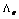and is related to specific conductance as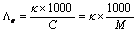(M is Molarity of the solution)

where C is the concentration in gram equivalent per litre (or Normality). This term has earlier been quite frequently used. Now it is replaced by molar conductance. The units of equivalent conductance are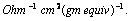Experimental measurement of conductance

(i) The conductance of a solution is reciprocal of the resistance, therefore, the experimental determination of the conductance of a solution involves the measurement of its resistance.

(ii) Calculation of conductivity: We have seen that conductivity (k)is reciprocal of resistivity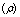, i.e.,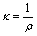and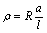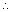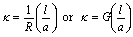where G is the conductance of the cell, l is the distance of separation of two electrodes having cross section area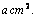The quantity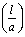is called cell constant and is expressed in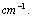Knowing the value of cell constant and conductance of the solution, the specific conductance can be calculated as,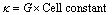i.e.,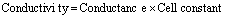## NEET & AIIMS Exam Sample Papers

 AIIMS SAMPLE PAPERS View More NEET SAMPLE PAPERS View More